## Selina Concise Mathematics Class 10 ICSE Solutions Chapter 9 Matrices Ex 9D

These Solutions are part of Selina Concise Mathematics Class 10 ICSE Solutions. Here we have given Selina Concise Mathematics Class 10 ICSE Solutions Chapter 9 Matrices Ex 9D.

Other Exercises

Question 1.
Find x and y, if: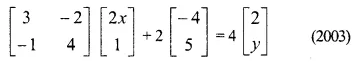Solution: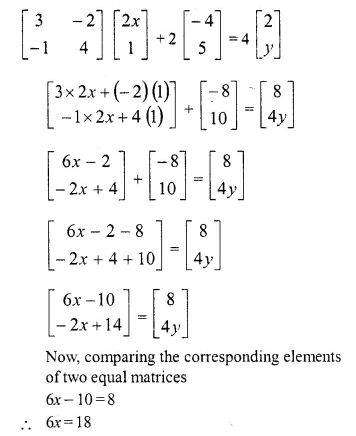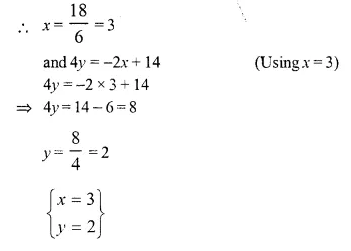Question 2.
Find x and y, if :Solution: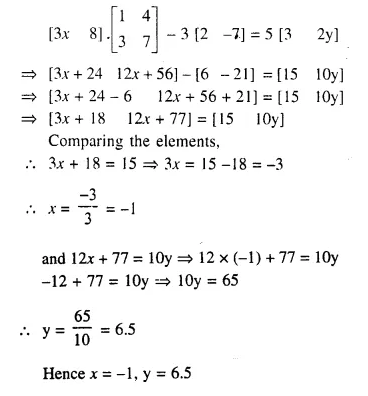Question 3.Solution: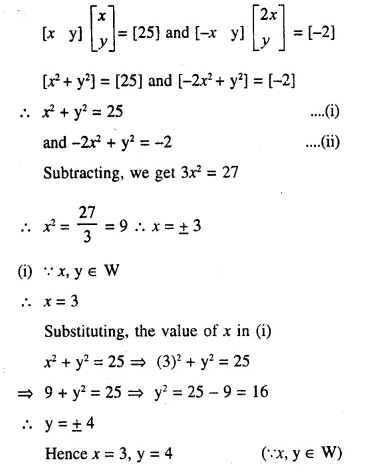Question 4.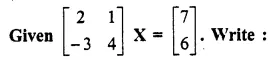(i) the order of the matrix X
(ii) the matrix X.
Solution: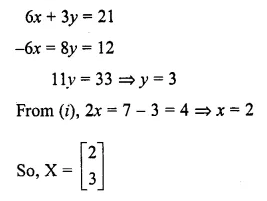Question 5.
Evaluate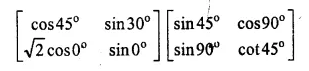Solution: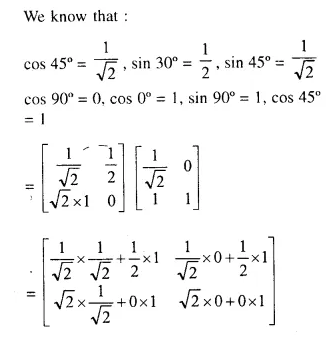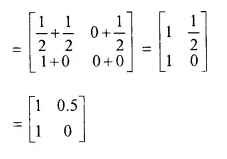Question 6.Solution: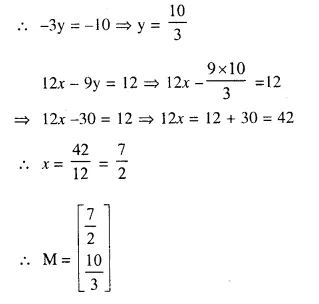Question 7.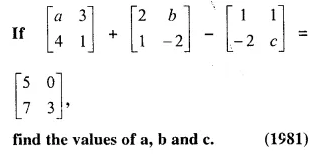Solution: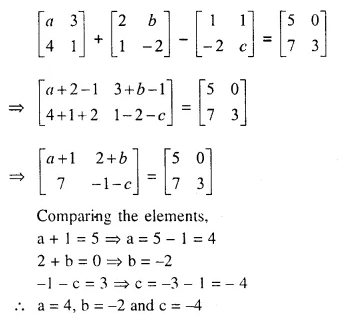Question 8.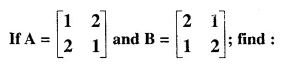(i) A (BA)
(ii) (AB) A
Solution: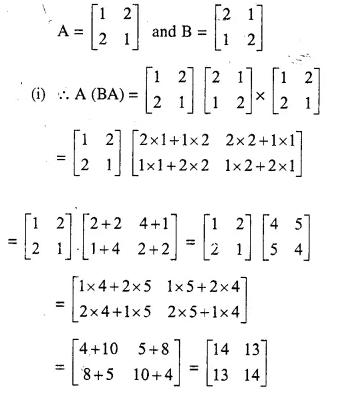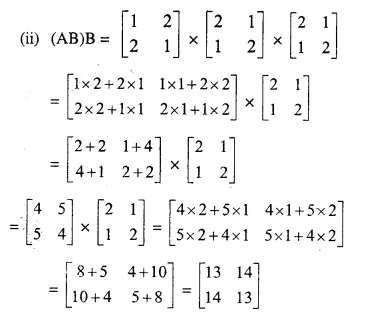Question 9.
Find x and y, if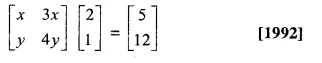Solution: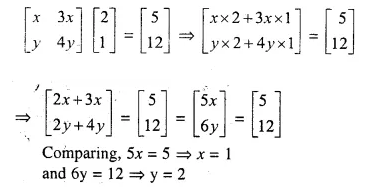Question 10.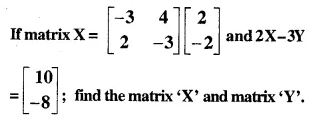Solution: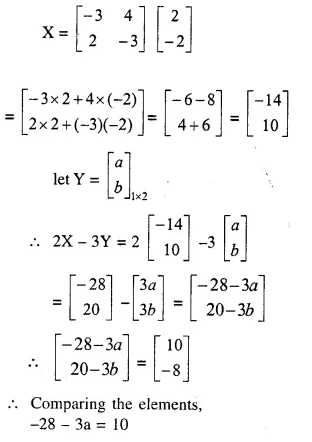Question 11.Solution: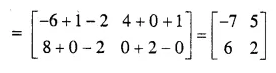Question 12.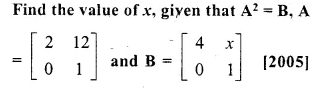Solution: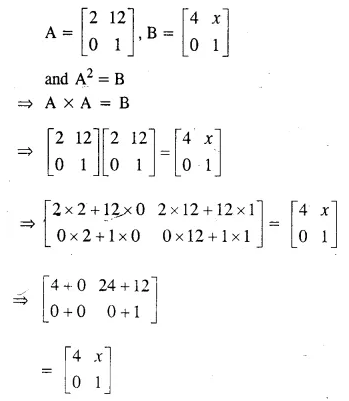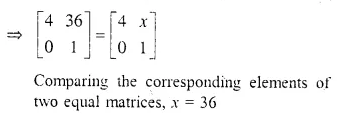Question 13.Solution: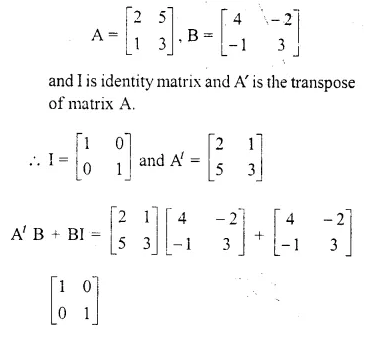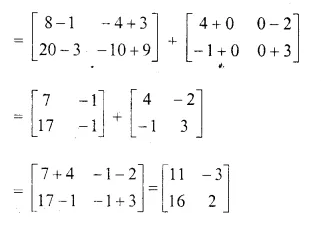Question 14.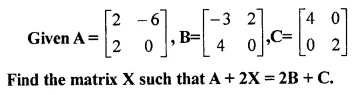Solution: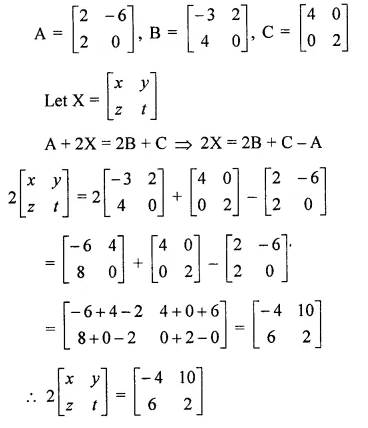Question 15.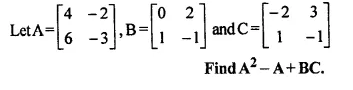Solution:Question 16.Solution: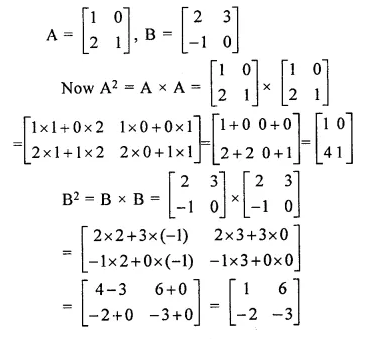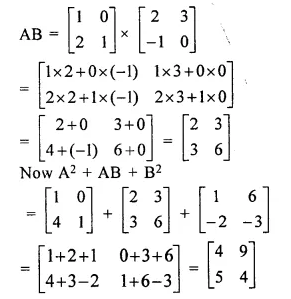Question 17.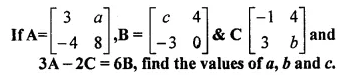Solution: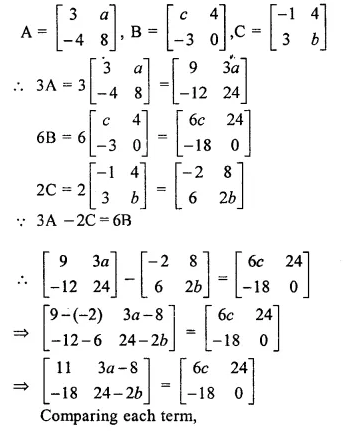Question 18.Solution: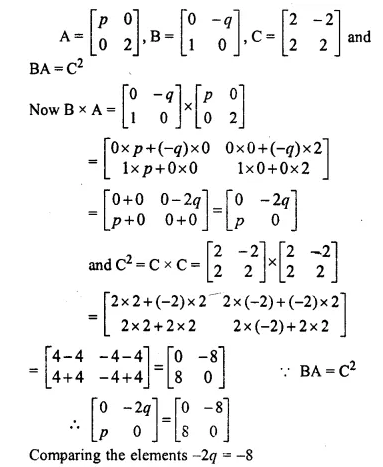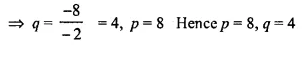Question 19.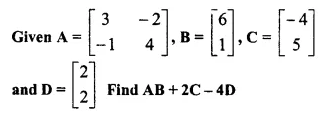Solution: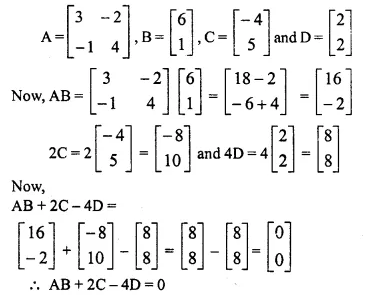Question 20.Solution: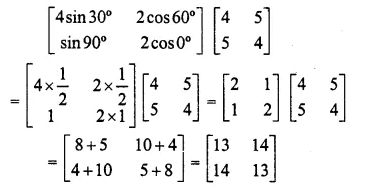Question 21.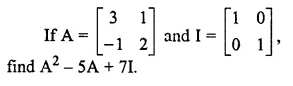Solution: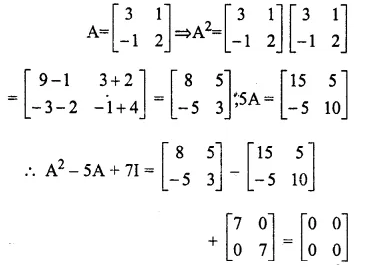Question 22.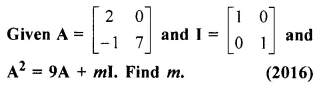Solution: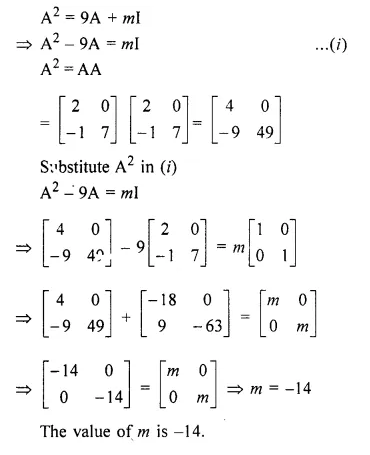Question 23.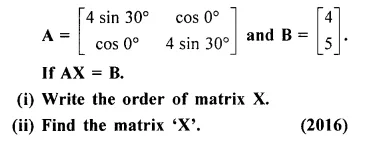Solution:
(i) Order of matrix A is 2 x 2
Order of matrix B is 2 x 1
Order of matrix X is 2 x 1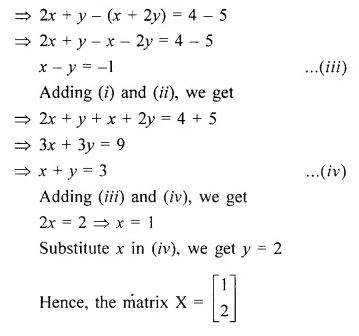Hope given Selina Concise Mathematics Class 10 ICSE Solutions Chapter 9 Matrices Ex 9D are helpful to complete your math homework.

If you have any doubts, please comment below. Learn Insta try to provide online math tutoring for you.